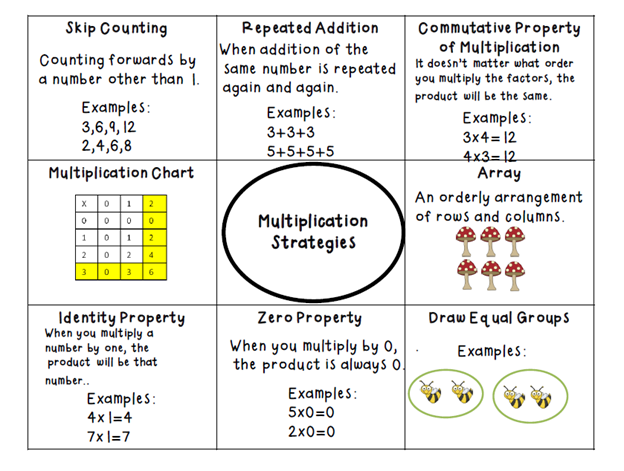﻿ Math 3-5

### School Closure Activities

Math 3-5Expanded Form/Place Value, Open Number Line and Adding in Chunks

Addition and Subtraction- Break Apart Strategy by Place Value

Subtraction

Compensating and Subtracting Strategy

Multiplication

Compensating with multiplication facts

Area Model for multiplication
2- digit by 1- digit

3- digit by 1, 2-digit by 2-digit and 3-digit by 2-digit

Break Apart Strategy

Break Apart Division
Basic Multiplication Strategies for Grade 3